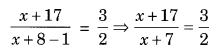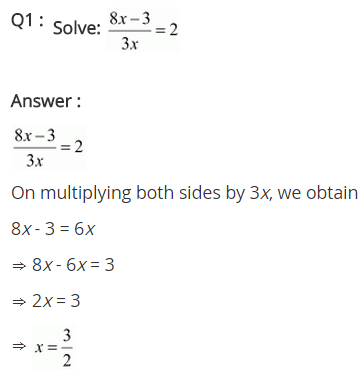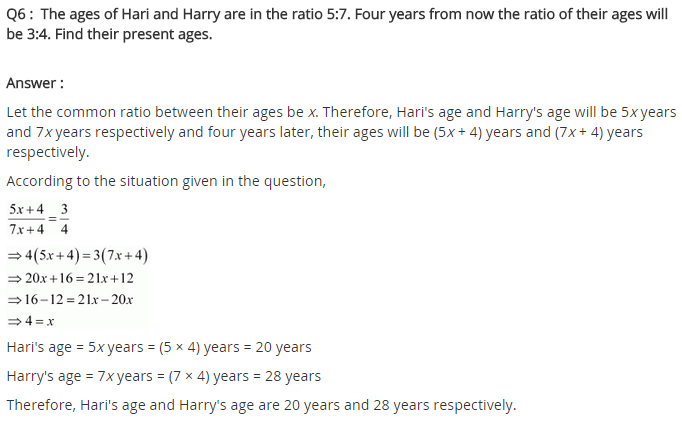# NCERT Solutions for Class 8 Maths Chapter 2 Linear Equations in One Variable Ex 2.6

NCERT Solutions for Class 8 Maths Chapter 2 Linear Equations in One Variable Ex 2.6

### NCERT Solutions for Class 8 Maths Chapter 2 Linear Equations in One Variable Exercise 2.6

Solve the following equations.
Ex 2.6 Class 8 Maths Question 1.Ex 2.6 Class 8 Maths Question 2.Ex 2.6 Class 8 Maths Question 3.Ex 2.6 Class 8 Maths Question 4.Ex 2.6 Class 8 Maths Question 5.Ex 2.6 Class 8 Maths Question 6.
The ages of Hari and Harry are in the ratio 5 : 7. Four years from now the ratio of their ages will be 3 : 4. Find their present ages.
Solution:
Let the present ages of Hari and Harry be 5x years and 7x years respectively.
After 4 years Hari’s age will be (5x + 4) years and Harry’s age will be (7x + 4) years.Hence the present ages of Hari and Harry are 5 × 4 = 20years and 7 × 4 = 28years respectively.

Ex 2.6 Class 8 Maths Question 7.
The denominator of a rational number is greater than its numerator by 8. If the numerator is increased by 17 and the denominator is decreased by 1, the number obtained is 3/2. Find the rational number.
Solution:
Let the numerator of the rational number be x.
Denominator = (x + 8)
As per the conditions, we have⇒ 2(x + 17) = 3(x + 7) (Cross-multiplication)
⇒ 2x + 34 = 3x + 21 (Solving the bracket)
⇒ 2x – 3x = 21 – 34 (Transposing 3x to LHS and 34 to RHS)
⇒ -x = -13
⇒ x = 13
Thus, numerator = 13
and denominator = 13 + 8 = 21
Hence the rational number is 13/21.## SabDekho

The Complete Educational Website